Home > ACC7 > Chapter cc38 > Lesson cc38.2.3 > Problem8-89

8-89.
1. In the table below, write each power of 10 as a decimal and as a fraction. Homework Help ✎

1. Describe how the decimals and fractions change as you progress down the table.

2. How would you tell someone how to write 10−12 as a fraction? You do not have to write the actual fraction.

 Power of 10 Decimal Form Fraction Form 100 10–1 0.1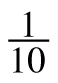10–2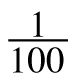10–3 10–4 10–5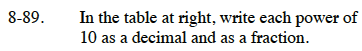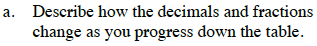100 = 1
10−2 = 0.01

Do you notice any patterns? Try to fill in the rest of the table on your own.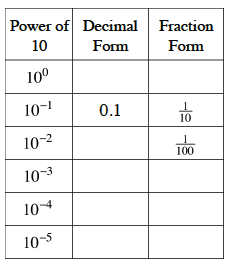Notice that the decimal point moves to the left one spot as the value of the exponent decreases, while the denominator in the fraction form increases by a multiple of 10.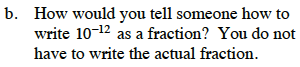From the table, you see that the magnitude of the exponent corresponds to the number of zeros in the denominator of the fraction form of the number.

Therefore the fraction form of this number would have 1 over a denominator of 1 with 12 zeros following.